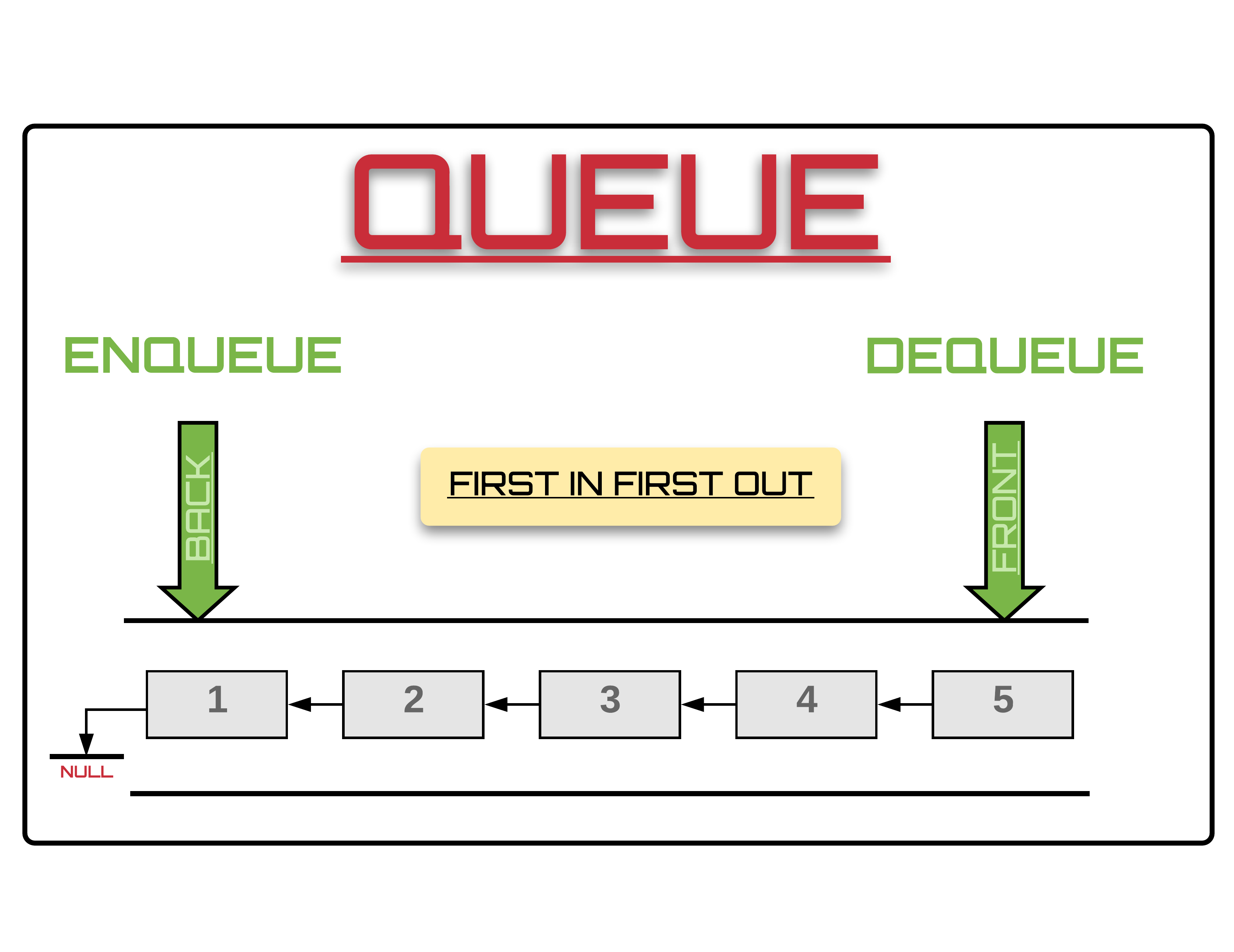Options
All
• Public
• Public/Protected
• All

# Class Queue<T>

## Queue (FIFO - first-in-first-out)

In computer science, a queue is a collection of entities that are maintained in a sequence and can be modified by the addition of entities at one end of the sequence and removal from the other end of the sequence. By convention, the end of the sequence at which elements are added is called the back or rear of the queue and the end at which elements are removed is called the head or front of the queue, analogously to the words used when people line up to wait for goods or services. The operation of adding an element to the rear of the queue is known as enqueue, and the operation of removing an element from the front is known as dequeue. Other operations may also be allowed, often including a peek or front operation that returns the value of the next element to be dequeued without dequeuing it.source: dev.to

### Complexity

Algorithm Average Worst case
Access `O(n)` `O(n)`
Search `O(n)` `O(n)`
Insert `O(1)` `O(1)`
Delete `O(1)` `O(1)`
- - -
Space `O(n)` `O(n)`

### Reference

``````import { Queue } from '@pencroff/ts-algorithms/dist/structure/linked';
const q = new Queue([1, 2, 3])``````

• Queue

## Constructors

### constructor

• new Queue(initArr?: T[]): Queue
• Queue constructor

#### Parameters

• ##### Optional initArr: T[]

initial data as array

## Accessors

### length

• get length(): number

## Methods

### __@iterator

• __@iterator(): Iterator<T>
• Queue iterator for iteration across all nodes

Complexity: O(n)

### clear

• clear(): void

• dequeue(): T

### enqueue

• enqueue(value: T): void
• Add new value to Queue tail

Complexity: O(1)

### has

• has(value: T): boolean
• Determines whether a value is in the Queue

Complexity: O(n)

• peek(): T

### toArray

• toArray(): T[]